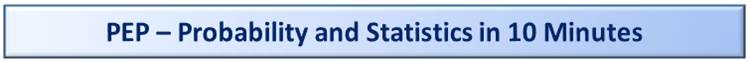Looking at Data in 10 Minutes#1

Overview

This is Part 1 of 3 for what you might find in a typical Intro to Probability and Statistics course.

This covers Mean, Median, Mode, Variance and Standard Deviation, Frequency Distributions and Histograms

Click this link for Part 2: Probability in 10 Minutes

Click this link for Part 3: Statistics in 10 Minutes

Who Is This For?

Someone without any prior experience, such as a college freshman about to take her first Statistics class.

Key Goals for Each of the 3 Parts

1) Introduce the Concepts, Terminology and Symbols that would be covered in a typical Intro to Statistics course.

2) Provide an intuitive understanding of things versus just providing formulas.

3) Present the information step-by-step in the best order for learning.

Looking at Data Part 1: Mean, Median and Mode

1) Mean: This is short for the Arithmetic Mean#2.

1.1) The Mean is the average of a set of numbers#3.

1.2) The formula is to sum up all values and divide by the total number of values#4.

1.3) The number of values is typically written as N for ‘Number’.  Uppercase ‘N’.

1.4) We’ll write out the values as x1 for the first value, x2 for the second value and so on, all the way up to xn for the last value with a lowercase ‘n’.

1.5) The Mean is typically written using Greek letter mu, which is µ

1.6) The uppercase Greek letter sigma which looks like this ∑ is typically used to mean ‘the sum of’.

1.7) We will therefore write the formula for the Mean as:

µ = x1 + x2 + x3 + ... + xn   / N

or as:

µ = ∑x / N

1.8) As an example, the Mean of these 3 numbers is 105.333333:

Number 1) 110

Number 2) 98

Number 3) 108

µ = (110 + 98 + 108) / 3

µ = 105.333333

1.9) The Mean of the values on a 6-sided die (singular of dice) is:

µ = (1 + 2 + 3 + 4 + 5 + 6) / 6

µ = 3.5

1.10) Observe that the mean does not need to be one of the source numbers. The Mean of 3.5 is not one of the vales on a die.

1.11) For the Mean, the order of the numbers does not matter.

The Arithmetic Mean of this set of numbers:

110, 98, 108

Is the same as the Arithmetic Mean of this (same) set of numbers (written in a different order):

98, 108, 110

1.12) Excel function to use is ‘AVERAGE’

2) Median:  This is the middle number in a set of numbers.  This requires that you order the numbers from smallest to largest.

2.1) For example, for this set of 5 numbers, the Median is 8.

Number 1) 1

Number 2) 8

Number 3) 8

Number 4) 9

Number 5) 9

By comparison, the Mean is:

µ = (1 + 8 + 8 + 9 + 9) / 5

µ = 7

2.2) Since the Median is just the middle number, in this example the Median would still be 8.

Number 1) -101

Number 2) 2

Number 3) 8

Number 4) 55

Number 5) 999

In the prior example, the Mean was 7, which is pretty close to the Median of 8.  In this example, the Mean is intentionally very different to illustrate the point.  The Mean here is 192.6.

2.3) The Median is used instead of the Mean in cases where a small number of very large values (or very small values) can Skew things one way or another.

The most common example is looking at incomes, how much money people earn in a year.

For example, if you have a room of 11 people with 10 average earners and Elon Musk (one of the richest people in the world), the Arithmetic Mean of their incomes, which would include Elon’s, might be over a million dollars.  While their Median income, which is the income of the 6th person, ranked from least income to most income, might be \$50,000, well under the average of \$1m.

2.4) Excel function to use is ‘MEDIAN’

3) Mode:  This is the most common number in a set of numbers#5.

As with the Mean, the order of the numbers does not matter in determining the Mode.  Although ordering the numbers is not a requirement, we’ll present the numbers ordered from lowest to highest to make it easier to see the most common numbers.

3.1) For this example of 6 numbers, the Mode is 9.  The number 9 repeats 3 times, more than any number.

Number 1) 1

Number 2) 8

Number 3) 8

Number 4) 9

Number 5) 9

Number 6) 9

Alternately, we can write this set of numbers as a list like this:

1, 8, 8, 9, 9, 9

3.2) If there are two numbers that repeat the same number of times and especially if they are non-adjacent the set of numbers is sometimes called ‘Bimodal’.

For example, this set of 10 numbers is Bimodal with Modes of 2 and 9.

1, 2, 2, 2, 6, 8, 8, 9, 9, 9

3.3) Excel function to use is ‘MODE’.

Looking at Data Part 2: Variance and Standard Deviation

1) Variance:  A measure of variation or variability of a set of numbers.  This is a hugely important concept in many areas such as finance and accounting.

Examples:

1.1) In finance, a stock or that has a bond that range of values is considered less risky than one that has a larger range of values.#6

1.2) In manufacturing, if you need to make a metal bar exactly one meter long, a machine that makes them 0.999 to 1.001 meters long (small variation) will be better than one that makes them 0.98 to 1.02 meters long (larger variation).  This is especially important when you are making many pieces that need to fit together, like parts of a car.

2) Standard Deviation: This is another measure of variation or variability of something.  This is related to the Variance.  The Standard Deviation is defined as the square root of the variance.

3) In practice sometimes the Standard Deviation will be used and sometimes the Variance will be used.  For the examples above for finance and manufacturing, the measurement of variation are more commonly presented as Standard Deviations.  You might see the Variance used in some formulas in Statistics.

The point is to make sure which one you are working with in any particular context.  Of course, if you have one of them, you can easily get the other by either squaring the value or taking the square root, as appropriate.

4) Symbols#7:

4.1) Variance: The symbol is the lowercase Greek letter sigma squared: σ2

4.2) Standard Deviation: The symbol is the lowercase Greek letter sigma (without the squared): σ

5) Formulas

5.1) The Variance of a population is the Average of the Squared Deviations from the Mean.

We’ll write that out as#8:

σ2 = [(x1 - µ)2 + (x2 - µ)2 + (x3 - µ)2 + … + (xn - µ)2] / N

Remembering that:

x1 = the first value

xn = the last value.  Lowercase ‘n’

µ = the Mean, i.e., the Arithmetic Mean

N = the total number of values.  Uppercase ‘N’.

5.2) The Standard Deviation is just the square root of that.

We’ll write that as either this: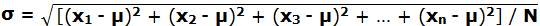Or like this, which is a bit easier to do on a computer:

σ = ( [(x1 - µ)2 + (x2 - µ)2 + (x3 - µ)2 + … + (xn - µ)2] / N )^1/2

6) Example:

Variance of a Die (Single of Dice).

Values are 1, 2, 3, 4, 5, 6.

N, the number of values, is 6.

Step 1) Figure out the Mean. As previously shown:

µ = (1 + 2 + 3 + 4 + 5 + 6) / 6

µ = 3.5

Step 2) Use this Formula for Variance.

σ2 = [(x1 3.5)2 + (x2 - 3.5)2 + (x3 - 3.5)2 + (x4 - 3.5)2 + (x5 - 3.5)2 + (x6 - 3.5)2] / 6

σ2 = [(1 3.5)2 + (2 - 3.5)2 + (3 - 3.5)2 + (4 - 3.5)2 + (5 - 3.5)2 + (6 - 3.5)2] / 6

σ2 = [(–2.5)2 + (–1.5) 2 + (–0.5)2 + (+0.5)2 + (+1.5)2 + (+2.5)2] / 6

σ2 = [6.25 + 2.25 + 0.25 + 0.25 + 2.25 + 6.25] / 6

σ2 = 17.5 / 6

σ2 = 2.916667

6.25

2.25

0.25

0.25

2.25

6.25

Step 3) And then take the square root to calculate the Standard Deviation.

σ = 2.916667^1/2

σ = 1.707825

7) Excel functions to use depending on your version of Excel are:

For Variance:

=VARP(A1:A6)

or

=VAR.P(A1:A6)

For Standard Deviation:

=STDEVP(A1:A6)

or

=STDEV.P(A1:A6)

Notes:

7.1) The ‘P’ in the function names is for Population. There are similarly named functions, e.g., STDEV (without the ‘P’) which will be used later when we discuss topics on Statistics.  The formulas are slightly different.#9

7.2) The Excel functions were given assuming that the data was located in Cells A1 to A6.  You’ll need to vary those values for your data as appropriate.

Looking at Data Part 3: Frequency Distributions

1) Frequency Distribution:  A table of data showing counts (frequencies) of numbers for single numbers or for a range.

2) Example

We’ll look at the count of the sum of 2 dice.  Each die has values 1 to 6 which allows for the sum ranging from 2 to 12.

We’ll use this notation (Dice1, Dice2) to show the values.  For example, if Dice1 is a 2 and Dice2 is a 5, that would look like this:

(2, 5)

Importantly, if Dice1 is 5 and Dice2 is 2, then that would look like this:

(5, 2)

In other words (2, 5) and (5, 2) are considered different, even though they both sum up to 7.  Using this approach there are 36 possible variations for 2 dice.

Here are the Sum of Two Dice with details shown for sums from 2 to 7:

Sums to 2: Frequency = 1

(1, 1)

Sums to 3: Frequency = 2

(1, 2), (2, 1)

Sums to 4: Frequency = 3

(1, 3), (3, 1), (2, 2)

Sums to 5: Frequency = 4

(1, 4), (4, 1), (2, 3), (3, 2)

Sums to 6: Frequency = 5

(1, 5), (5, 1), (2, 4), (4, 2), (3, 3)

Sums to 7: Frequency = 6

(1, 6), (6, 1), (2, 5), (5, 2), (3, 4), (4, 3)

Sums to 8: Frequency = 5

Sums to 9: Frequency = 4

Sums to 10: Frequency = 3

Sums to 11: Frequency = 2

Sums to 12: Frequency = 1

3) Classes:  Classes are the number of groupings for a Frequency Distribution.  Other terms for this concepts are ‘Ranges’ or ‘Buckets’.

For the prior example of the sum of two dice, there are 11 Classes, ranging from 2 to 12.

If we wanted, we would reduce the number of classes from 11 down to just 6, with values based on ranges like this:

Class1: Dice Sum to 1 to 2: Frequency = 1

Class2: Dice Sum to 3 to 4: Frequency = 5

Class3: Dice Sum to 5 to 6: Frequency = 9

Class4: Dice Sum to 7 to 8: Frequency = 11

Class5: Dice Sum to 9 to 10: Frequency = 7

Class6: Dice Sum to 11 to 12: Frequency = 3

See that if you sum up the frequencies, you get the same total of 36.

36 = 1 + 5 + 9 + 11 + 7 + 3

Looking at Data Part 4: Histogram

1) Histogram:  A graphical representation of a Frequency Distribution

2) Examples

Example 1

Based on the original example of the sum of 2 dice.

The Y-axis is the count.  The X-axis is the Class.

For example, see the bar in the lower left.  That shows that there is 1 case when dice sum to 2.  In the middle it shows there are 6 cases when dice sum up to 7.

See that the total number of possible sums of 2 dice is still 36 even when viewed as a graph.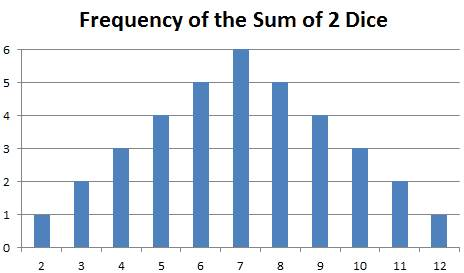Example 2

This is based on the 2nd example of having 6 Classes for the Sum of 2 Dice Rolls.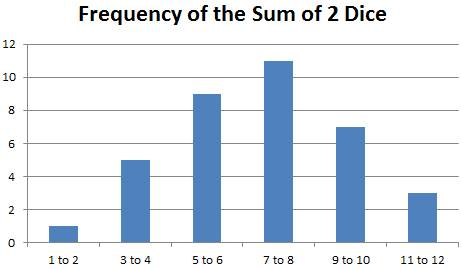3) Looking at Histograms, Mean, Median and Mode

Typical for Intro to Statistics classes you be asked to look at a Histogram and answer questions about the Mean, Median and Mode.

We’ll provide the frequencies here in table form (Frequency Distribution), though for a test you’ll likely just get the Histogram.#10

 Range Frequency 1 to 10 4 11 to 20 8 21 to 30 15 31 to 40 25 41 to 50 43 51 to 60 63 61 to 70 75 71 to 80 62 81 to 90 41 91 to 100 31

That totals to 367 different values:

367 = 4 + 8 + 15 + 25 + 43 + 75 + 62 + 41 + 31

Histogram: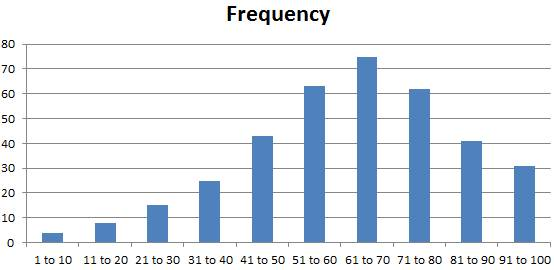Questions:

Q1) What is the Mode?

A1) This is typically the easiest to discern.  This is the number or range of numbers that has the highest frequency.  In this case, that is the range from 61 to 70, which has a total of 75 values within that range.

Q2) Which is higher, the Median or the Mean?

A2) This is a bit harder to figure out just by looking at the above Histogram.  We’ll show a trick on how to figure this out.

As a reminder, the Median is the middle value, ranked from smallest to largest.  There are 367 values in total, so the middle value would be the 184th number.  Although we are not going to use that directly here.

For the trick, we start by observing the values are skewed to the left.  Then we can create a smaller set of values that we can more easily wrap our heads around.

Let’s use these 5 value values:

1, 8, 8, 9, 9

That looks like this as a Histogram.  This is also skewed to the left though somewhat simplified.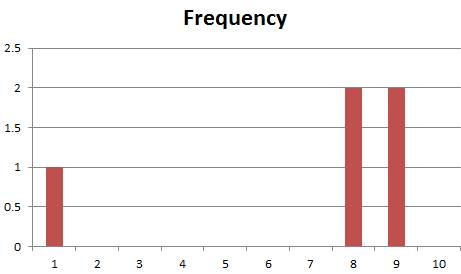The Median is 8, as that is the middle number, the third of the 5 numbers:

1, 8, 8, 9, 9

For the Mean, we have:

µ = (1 + 8 + 8 + 9 + 9) / 5

µ = 35 / 5

µ = 7

The answer in this case is the Mean is less than the Median, which will be true for Distributions that are skewed to the left.

For distributions that are skewed to the right, that would be reversed and the Mean would be higher than the Median.

Example data:

1, 1, 8, 8, 9

Median: 8

Mean: 5.4

The key here is that you may be asked this question just by looking at a Histogram and without having access to the underlying table of data.

Footnotes

#1) The title of this page was inspired by ‘Learn Python in 10 Minutes’ at:

#2) Another ‘mean’ is the ‘Geometric Mean’. That has a formula where you multiply all of the numbers and then take the square root.  For example, for these three numbers which represent the assumed returns on a stock for three years:

Year 1: 110%

Year 2: 98%

Year 3: 108%

We are writing this to show the ending value relative to the starting value, so by 110% we mean that the stock went up 10%.  And for 98%, we mean that the stock went down 2%.

This is called the ‘one plus’ approach, since we are adding one to the return.  i.e., take -2% and add one and get +98%.

This makes the math nicer for Geometric Means because we don’t need to worry about negative numbers, since we are assuming stock prices can’t go below zero.

For the above example, we get this Geometric Mean:

Geometric Mean = (110% * 98% * 108%)^1/3

Geometric Mean = (116.42400%)^1/3

Geometric Mean = 105.19962%

Compare that to the Arithmetic Mean of 105.33333%, which is just summing the numbers and dividing by 3.

#3) The Mean is the ‘Simple Average’ of a set of numbers.  There is another kind of average called the ‘Weighted Average’, which each of the values you are averaging is given a weighting other than 1/n.

#4) While it is not important for now, try to remember for later that the concept of the Mean (Arithmetic Mean) applies only when we have a set of numbers which represent the complete set of things.  The term for this is ‘Population’.  The average of a Population is known as the Mean and we use the symbol µ.

When we are working with a Sample of data, there is a different term used for the average and a different symbol.   The term is Sample Mean and the symbol is an x with a bar over it: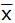Sample Mean =An example of a sample would be to pick 10 students at random from a class of 30 and take their average height.  The Sample Mean would be the average of the 10 selected students.  In the Statistics Part, we’ll show how to use Statistical Inference to Estimate the Population Mean of the overall population of 30 students and provide a range around it called a Confidence Interval where we can be 95% sure, based on some assumptions, that the true Population Mean of the 30 students is within that range.

#5) The Mode as a value is as a practical matter used far less than the Mean or the Median.  The Mean is by far the most used and so most important concept to know.

#6) For stocks, bond and commodities, finance professionals typically measure the change in values from one do to another when determining how risky a something is.  For simplicity, we’ll say they use the percentage change.  Finance professionals will use the term ‘Volatility’ to describe the Standard Deviation of the percent price change on an annualized basis.

Examples:

6.1) A stock that trades  \$100 one day, then \$110 the next and then \$105 on the third day has day-over-day percentage moves of up 10% and then down 4.5%.

That is considered less risky than a stock that trades like this:

6.2) \$4 on day 1, \$5 on day 2 and \$4.25 on day 3.   Those percentages are much higher.  25% up move from Day 1 to Day 2 and 15% down move from Day 2 to Day 3.

#7) While it is not important for now, try to remember for later that the symbols σ2 for Variance and σ for Standard Deviation are to be used only when talking about Population, meaning the complete set of numbers.  The term for this is ‘Population’.

When we are working with a Sample of data, there is a different symbol used.  For a Sample of Data, we use lowercase s for Standard Deviation and then square it for Variance.

Variance of a Sample: s2

Standard Deviation of a Sample: s

#8) This formula for Variance should be used only when working with a Population, meaning you know all of the possible values.  When dealing with a Sample of data from a bigger Population, there is a slightly different formula.  That will be discussed in the Statistics section.  As a comparison, the formulas for Arithmetic Mean (of a population) and Sample Mean are basically the same.  The formulas only diverge when we get to Population Variance versus Sample Variance.

8.1) Some may wonder why we take the square of the difference.  That is to get rid of negatives.  Suppose we just took the average of the differences for each value from the mean.  Without the squaring.  That would look like this using the single die as an example:

??? = [(x1 3.5) + (x2 - 3.5) + (x3 - 3.5) + (x4 - 3.5) + (x5 - 3.5) + (x6 - 3.5)] / 6

??? = [(1 3.5) + (2 - 3.5) + (3 - 3.5) + (4 - 3.5) + (5 - 3.5) + (6 - 3.5)] / 6

??? = [(–2.5) + (–1.5) + (–0.5) + (+0.5) + (+1.5) + (+2.5)] / 6

??? = 0 / 6

??? = 0

An average deviation of 0 (zero) would not make much sense.

An alternate approach might be to take the average of the absolute value of the differences.

Average Deviation = [abs(x1 3.5) + abs(x2 - 3.5) + abs(x3 - 3.5) + abs(x4 - 3.5) + abs(x5 - 3.5) + abs(x6 - 3.5)   ] / 6

Average Deviation = [abs(1 3.5) + abs(2 - 3.5) + abs(3 - 3.5) + abs(4 - 3.5) + abs(5 - 3.5) + abs(6 - 3.5)] / 6

Average Deviation = [2.5 + 1.5 + 0.5 + 0.5 + 1.5 + 2.5] / 6

Average Deviation = 9 / 6

Average Deviation = 1.5

A value of 1.5 is more reasonable.  That said, we won’t be saying anything more about the Average Deviation as it is rarely used relative to the Variance and the Standard Deviation.

#9) See this link for an explanation of the difference in the formulas for the Population Variance versus the Sample Variance.  Note: When we say ‘Sample Variance’ we really mean to say that we are using the data from a Sample to derive an Unbiased Estimator of Population Variance for the overall Population from which we took the samples.

#10) Looking at the Frequency Distribution and looking at the first class, which is for values ranging from 1 to 10, a note that we don’t know exactly what those values are.

They could be:

1, 1, 5, 6

Or

7, 8, 8, 9

Or non-whole numbers like

1.5, 6.5, 7.343, 8.9

All we know about them is that there are 4 of them and they are greater or equal to 1 and less than or equal to 10.